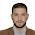## MATLAB and Simulink for Engineers (Oxford Higher Education)

Based on the latest version of MATLAB (2010a), the book begins with an introduction to MATLAB programming describing the MATLAB toolbar and SIMULINK toolboxes. Further the various MATLAB operators, functions, and graphics are discussed in detail. Applications of SIMULINK and MATLAB in electrical engineering, electrical machines & power system projects, simulation of rectifiers, inverters, choppers, and cycloconverters is presented in detail. Applications in computer science, communication and mechanical engineering is also discussed. Finally a chapter on recent developments in this area covers topics such as the matrix converter, modulation techniques, and PWM rectifiers.

The book provides numerous MATLAB programs tested and run for various projects for different disciplines in engineering. The programs, complete with their outputs, through various screen shots of the MATLAB windows help readers familiarise themselves with the software.

1.